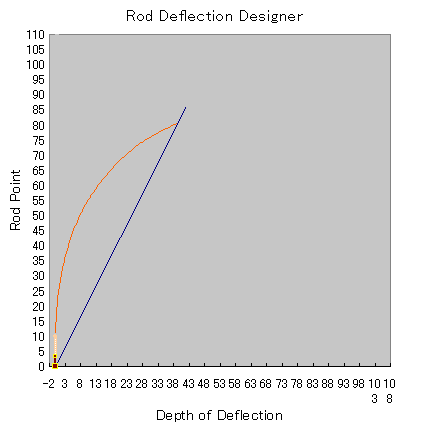﻿ DynaRod :: Rod ActionIntroduction Download User Guide About

Rod Action has several connotations. The strength of reflection, speed of reflex, depth of deflection, and which part of a rod bends more, etc., etc.. The diversity of understanding is making the word "Rod Action" vague and gives an image of a rod wrongly to people.

Max Rod Craft challenges to this situation.

Here, the following three factors define Rod Action;

Under a certain assumptions,

• Rod Angle - The measure of stiffness/softness of entire rod length
• Tip Top Tangent Angle - Aggregate angle which is the accumulation of bend angle of each 1" section
• Action Genome - Pattern of deflection shape when the rod is in action

### Rod Angle

The angle made between the rod axis line (the extension of grip) and the line connecting the tip top and the butt end, when a rod deflects. It is same when the rod is held horizontally.

When the Rod Angle is the less, the rod is the stiffer, and the Rod Angle is the wider, the rod is the softer. This is the measure of stiffness/softness of a rod under a certain (or a standardized) assumption.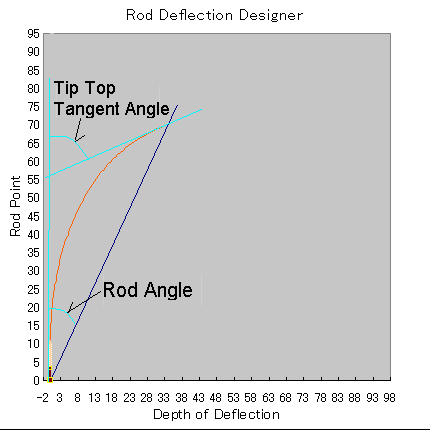t is far more understandable to refer to the Rod Angle than saying Fast or Slow.

A certain standardized assumptions mean the following;  when measuring by actual experimentation, we can hang a certain amount of weight at the tip top. (not described here)

Instead of actually experimenting the deflection with a weight, we can calculate the Rod Angle in a second using personal computer today.  Of-course the result can be compared with the actual experimentation.

Standard Assumptions:

• Rod Speed:        4G speed.  The speed where the rod receives 4 times of gravity acceleration.
• Rod Movement:  Translated.  Move a vertically held rod in parallel.
• Calculation:         by DynaRod.  DynaRod is the software which is developed by Max Satoh
• The load of fly line:    The list below is defined as the standard.
•     #3    30 feet
•     #4    40 feet
•     #5    50 feet
•     #6    60 feet
•     #7    70 feet
•     #8    80 feet
•     #9    90 feet
 Rod Angle stiffness/softness acronym -15      degrees Very Hard VH 15-20  degrees Hard H 20-25  degrees Medium Hard MH 25-30  degrees Medium M 30-35  degrees Medium Soft MS 35-40  degrees Soft S 40-      degrees Very Soft VS

### Tip Top Tangent Angle (or just Top Angle)

The Angle made between a vertical line and tangent of the 1st 1" of the tip top, when an entire rod bend is measured in 1" interval.

According to the physical relationship, this angle represent the total of each bend angle for each 1" section of the rod.  The 1" section's bend angle is measured against the angle of 1" prior section.  So Tip Top Tangent Angle can be said as the total of rod bends or magnitude of bend angle.

On the graph below, the accumulation of y value from right side (butt side) toward left (tip side) becomes the angle of tangent at the tip top section. (as shown in the graph above).  This is the physics.  When we shift the entire line upward, the Tip Top Tangent Angle becomes larger, and it means that the rod will bend a little more deeper, for larger Rod Angle.  How to distribute the total angle value (Tip Top Tangent Angle) over the entire rod varies the shape of rod deflection  This is the Action Genome.

### Action Genome (Action Pattern)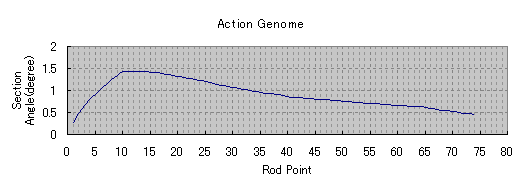This action genome graph can be understood that it shows the exact bend angle from previous 1" section at each rod point with 1" interval.  The higher part of the y value means it bends more at the rod point.

This curve has same nature to Stress Curve which Everett Garrison introduced.   The pattern how much to bend at which part is called as Action Genome, that is the DNA of rod action.  Same type genome will generate same type rod deflection.

Action Genome and Rod Deflection Graph (shown above) are used together as the rod design tool.

The benefits of this design method are;

•  we can see both graphs at the same time and both moves together on the same surface
•  we can reverse calculate the dimension from the action genome

These are packaged in DynaRod as Deflection Designer tool, which is developed by  Excel.

## Basic Action Genome Patterns

Let's see the relationships between Action Genome and corresponding Rod Deflection graph, as Action Genome Patterns.

Each case are equally for Rod Angle = 18 degrees.  So they all belongs to Hard rod category.

Other than these three, Action Genome can generate as many type of deflection shape.  In other words, Action Genome can create whatever type of rod actions and corresponding tapers.

### Progressive Action Genome Pattern (Top Angle = 60 degrees)

From butt to tip, the degree of bend increases.  So called Tip Action.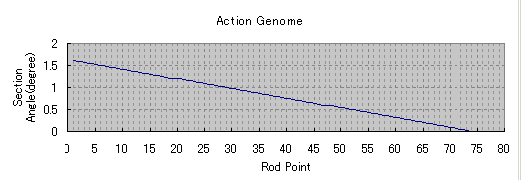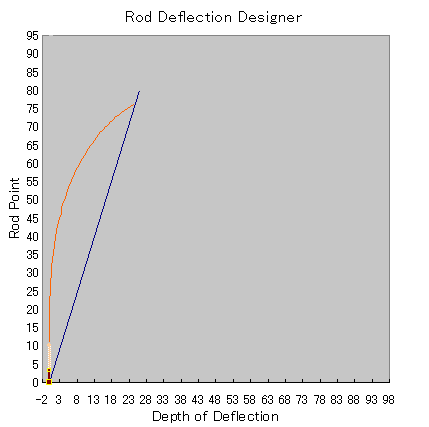### Flat Action Genome Pattern (Top Angle = 40 degrees)

Every part of the rod bends equally.  So called Middle Action.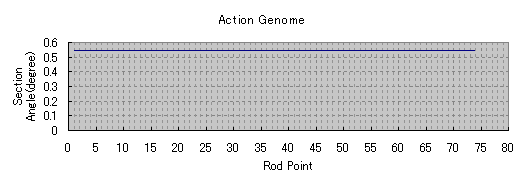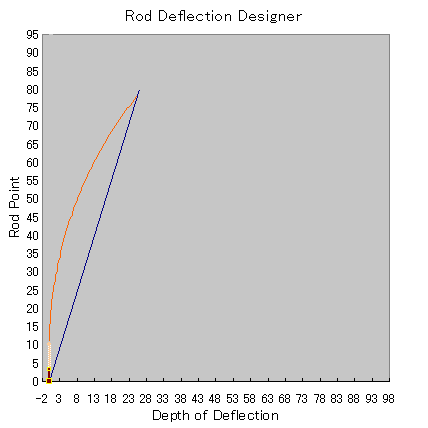### Parabolic Action Genome Pattern (Top Angle =30 degrees)

The more bend at butt side and gradually eliminate the bend toward tip top.  So called Butt Action.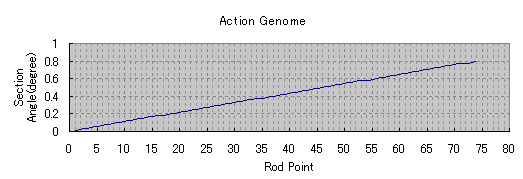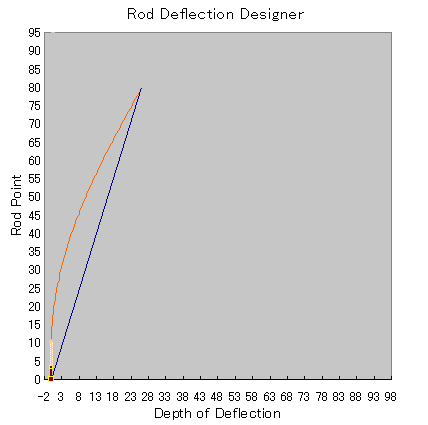### Example of Classic Rod

Below is the Action Genome of Dickerson 8013 8 feet 3 pieces #5wt.

Rod Angle=26 degrees (stiffness=Medium), Top Angle=65 degrees.   Action Genome Pattern is for Progressive with modification at the center part.   This graph is made under the assumption that  50 feet of WF#5 line is thrown in Translated cast with 4G speed.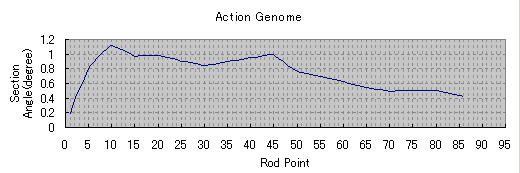The jag of the graph line up and down seems caused by the rod design method in old days.  They made refinement of taper by trial and error.   By eliminating dimensions over there and here, this rod seems to be produced.  Again, this taper seems not the original of Dickerson taper itself but seems to be taken for 5" interval Planing Form  in later days as the turning point of the curve matches to each 5" station.

This Action Genome basically belongs to Progressive Pattern but the author may have needed to flex at the center part of the rod a little more.  The resulted rod deflection would be like below.